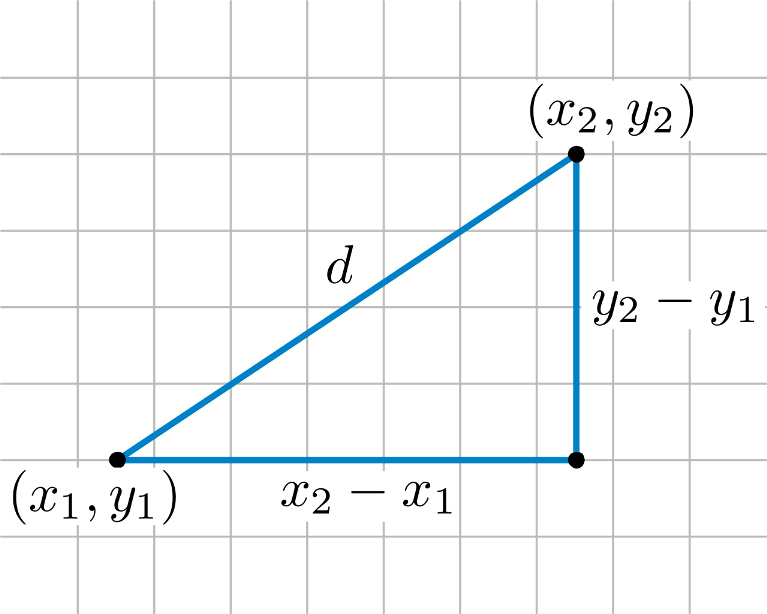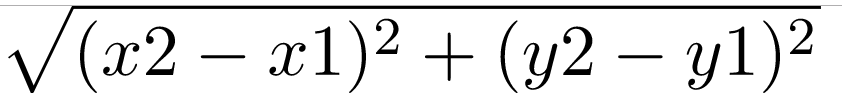×

Search anything:

# Distance between two points in 2D space

#### Algorithms List of Mathematical AlgorithmsGet this book -> Problems on Array: For Interviews and Competitive Programming

Reading time: 30 minutes | Coding time: 10 minutes

Distance between two points is calculated by creating a right-angled triangle using the two points.The line between the two points is the hypotenuse (the longest side, opposite to the 90° angle). Lines drawn along the X and Y-axes complete the other sides of the triangle, whose lengths can be found by finding the change in X and change in Y between the two points.We will use the Distance formula derived from Pythagorean theorem.

Diagram :The formula for distance between two point (x1, y1) and (x2, y2) is### Algorithm

step 1 : Take a two points as an input from an user.
step 2 : Calculate the difference between the corresponding X-cooridinates i.e : X2 - X1 and Y-cooridinates i.e : Y2 - Y1 of two points.
step 3 : Apply the formula derived from Pythagorean Theorem
i.e : sqrt((X2 - X1)^2 + (Y2 - Y1)^2)
step 4 : Exit.

### Implementation

``````#include <iostream>
#include <cmath>
class DistancebwTwoPoints
{
public:
void getCoordinates();
double calculateDistance();

private:
double x1_, y1_, x2_, y2_;

};

void DistancebwTwoPoints::getCoordinates()
{
std::cout << "\nEnter the Cooridinates of Two points ";
std::cout << "\nEnter X1 : ";
std::cin >> x1_;
std::cout << "\nEnter Y1 : ";
std::cin >> y1_;
std::cout << "\nEnter X2 : ";
std::cin >> x2_;
std::cout << "\nEnter Y2 : ";
std::cin >> y2_;
}

double DistancebwTwoPoints::calculateDistance()
{
return sqrt(pow(x2_ - x1_, 2) + pow(y2_ - y1_, 2));
}

int main()
{
DistancebwTwoPoints d;
d.getCoordinates();
std::cout << "\nDistance between given two points is " << d.calculateDistance() << " units.";
}
``````

### Complexity

The time and space complexity to calculate Distance between two points :

Assuming arithmetic operations (like multiplication and square root) take constant time O(1):

• Worst case time complexity: `Θ(1)`
• Average case time complexity: `Θ(1)`
• Best case time complexity: `Θ(1)`
• Space complexity: `Θ(1)`
Actual time complexity:

Considering numeric input N and multiplication takes O((log(N))^2) time:

• Worst case time complexity: `Θ((log(N))^2)`
• Average case time complexity: ` Θ((log(N))^2)`
• Best case time complexity: ` Θ((log(N))^2)`
• Space complexity: `Θ(1)`

A number N has log(N) digits and the most common way of multiplication takes O(D^2) time complexity where D is the number of digits = log N.

Square root is usually calculated using Newton's method which takes the same complexity as that of the multiplication algorithm used. Hence, the overall time complexity of calculating distance between two points is ` Θ((log(N))^2)`.#### Piyush Rajendra Chaudhari

A man who wants to make an impact on the lives of people through the code.

Distance between two points in 2D space